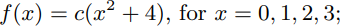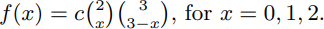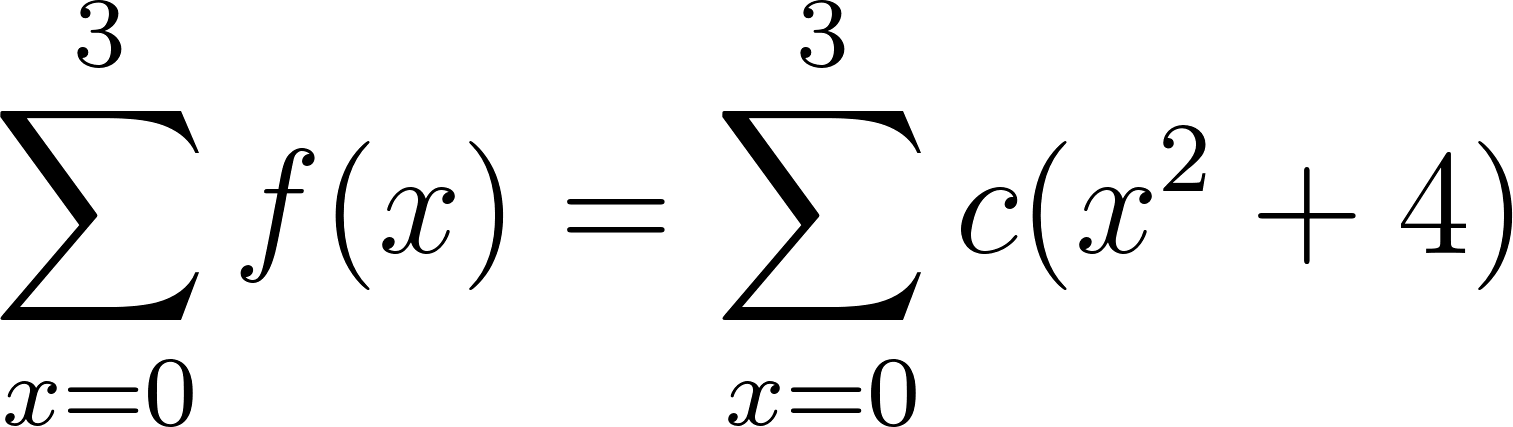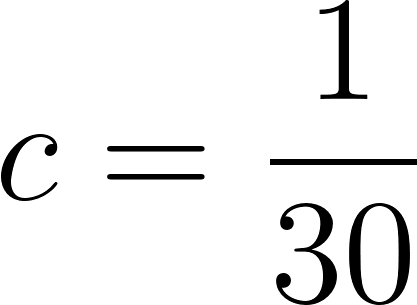×
Get Full Access to Probability And Statistics For Engineers And The Scientists - 9 Edition - Chapter 3 - Problem 3.5
Get Full Access to Probability And Statistics For Engineers And The Scientists - 9 Edition - Chapter 3 - Problem 3.5

×

# Solved: Determine the value c so that each of theISBN: 9780321629111 32

## Solution for problem 3.5 Chapter 3

Probability and Statistics for Engineers and the Scientists | 9th Edition

• Textbook Solutions
• 2901 Step-by-step solutions solved by professors and subject experts
• Get 24/7 help from StudySoup virtual teaching assistantsProbability and Statistics for Engineers and the Scientists | 9th Edition

4 5 1 354 Reviews
29
3
Problem 3.5

Determine the value c so that each of the following functions can serve as a probability distribution of the discrete random variable X: (a) f(x)=c(x2 + 4), for x =0 ,1,2,3; (b) f(x)=c2 x 3 3x, forx =0 ,1,2.

Step-by-Step Solution:

Problem 3.5

Determine the value c so that each of the following functions can serve as a probability distribution of the discrete random variable X:

(a)(b)Step by Step Solution

Step 1 of 2

(a)

Given,To determine the value c we have to add the values of function for x = 0 to 3.Therefore,Step 2 of 2

##### ISBN: 9780321629111

Unlock Textbook Solution